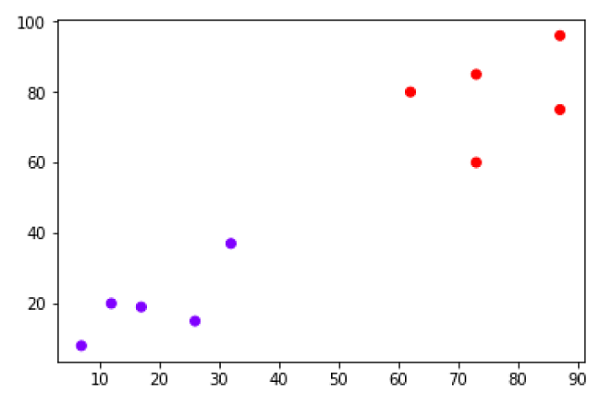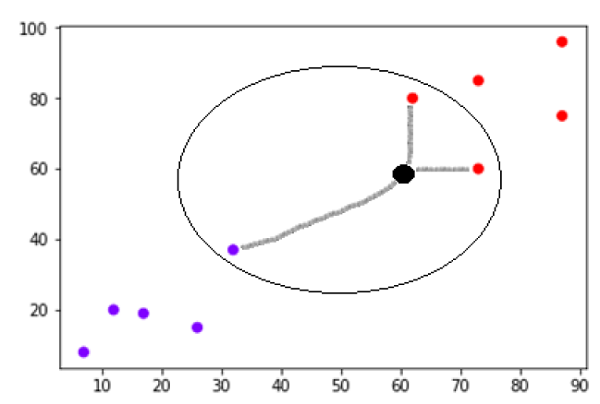# KNN Algorithm - Finding Nearest Neighbors

## Introduction

K-nearest neighbors (KNN) algorithm is a type of supervised ML algorithm which can be used for both classification as well as regression predictive problems. However, it is mainly used for classification predictive problems in industry. The following two properties would define KNN well −

• Lazy learning algorithm − KNN is a lazy learning algorithm because it does not have a specialized training phase and uses all the data for training while classification.

• Non-parametric learning algorithm − KNN is also a non-parametric learning algorithm because it doesn’t assume anything about the underlying data.

## Working of KNN Algorithm

K-nearest neighbors (KNN) algorithm uses ‘feature similarity’ to predict the values of new datapoints which further means that the new data point will be assigned a value based on how closely it matches the points in the training set. We can understand its working with the help of following steps −

• Step 1 − For implementing any algorithm, we need dataset. So during the first step of KNN, we must load the training as well as test data.

• Step 2 − Next, we need to choose the value of K i.e. the nearest data points. K can be any integer.

• Step 3 − For each point in the test data do the following −

3.1 − Calculate the distance between test data and each row of training data with the help of any of the method namely: Euclidean, Manhattan or Hamming distance. The most commonly used method to calculate distance is Euclidean.

3.2 − Now, based on the distance value, sort them in ascending order.

3.3 − Next, it will choose the top K rows from the sorted array.

3.4 − Now, it will assign a class to the test point based on most frequent class of these rows.

• Step 4 − End

### Example

The following is an example to understand the concept of K and working of KNN algorithm −

Suppose we have a dataset which can be plotted as follows −Now, we need to classify new data point with black dot (at point 60,60) into blue or red class. We are assuming K = 3 i.e. it would find three nearest data points. It is shown in the next diagram −We can see in the above diagram the three nearest neighbors of the data point with black dot. Among those three, two of them lies in Red class hence the black dot will also be assigned in red class.

## Implementation in Python

As we know K-nearest neighbors (KNN) algorithm can be used for both classification as well as regression. The following are the recipes in Python to use KNN as classifier as well as regressor −

## KNN as Classifier

import numpy as np
import matplotlib.pyplot as plt
import pandas as pd


path = "https://archive.ics.uci.edu/ml/machine-learning-databases/iris/iris.data"


Next, we need to assign column names to the dataset as follows −

headernames = ['sepal-length', 'sepal-width', 'petal-length', 'petal-width', 'Class']


Now, we need to read dataset to pandas dataframe as follows −

dataset = pd.read_csv(path, names=headernames)

slno. sepal-length sepal-width petal-length petal-width Class
0 5.1 3.5 1.4 0.2 Iris-setosa
1 4.9 3.0 1.4 0.2 Iris-setosa
2 4.7 3.2 1.3 0.2 Iris-setosa
3 4.6 3.1 1.5 0.2 Iris-setosa
4 5.0 3.6 1.4 0.2 Iris-setosa

Data Preprocessing will be done with the help of following script lines −

X = dataset.iloc[:, :-1].values
y = dataset.iloc[:, 4].values


Next, we will divide the data into train and test split. Following code will split the dataset into 60% training data and 40% of testing data −

from sklearn.model_selection import train_test_split
X_train, X_test, y_train, y_test = train_test_split(X, y, test_size=0.40)


Next, data scaling will be done as follows −

from sklearn.preprocessing import StandardScaler
scaler = StandardScaler()
scaler.fit(X_train)
X_train = scaler.transform(X_train)
X_test = scaler.transform(X_test)


Next, train the model with the help of KNeighborsClassifier class of sklearn as follows −

from sklearn.neighbors import KNeighborsClassifier
classifier = KNeighborsClassifier(n_neighbors=8)
classifier.fit(X_train, y_train)


At last we need to make prediction. It can be done with the help of following script −

y_pred = classifier.predict(X_test)


Next, print the results as follows −

from sklearn.metrics import classification_report, confusion_matrix, accuracy_score
result = confusion_matrix(y_test, y_pred)
print("Confusion Matrix:")
print(result)
result1 = classification_report(y_test, y_pred)
print("Classification Report:",)
print (result1)
result2 = accuracy_score(y_test,y_pred)
print("Accuracy:",result2)


### Output

Confusion Matrix:
[[21 0 0]
[ 0 16 0]
[ 0 7 16]]
Classification Report:
precision      recall       f1-score       support
Iris-setosa       1.00        1.00         1.00          21
Iris-versicolor   0.70        1.00         0.82          16
Iris-virginica    1.00        0.70         0.82          23
micro avg         0.88        0.88         0.88          60
macro avg         0.90        0.90         0.88          60
weighted avg      0.92        0.88         0.88          60

Accuracy: 0.8833333333333333


## KNN as Regressor

import numpy as np
import pandas as pd


path = "https://archive.ics.uci.edu/ml/machine-learning-databases/iris/iris.data"


Next, we need to assign column names to the dataset as follows −

headernames = ['sepal-length', 'sepal-width', 'petal-length', 'petal-width', 'Class']


Now, we need to read dataset to pandas dataframe as follows −

data = pd.read_csv(url, names=headernames)
array = data.values
X = array[:,:2]
Y = array[:,2]
data.shape

output:(150, 5)


Next, import KNeighborsRegressor from sklearn to fit the model −

from sklearn.neighbors import KNeighborsRegressor
knnr = KNeighborsRegressor(n_neighbors=10)
knnr.fit(X, y)


At last, we can find the MSE as follows −

print ("The MSE is:",format(np.power(y-knnr.predict(X),2).mean()))


### Output

The MSE is: 0.12226666666666669


## Pros and Cons of KNN

### Pros

• It is very simple algorithm to understand and interpret.

• It is very useful for nonlinear data because there is no assumption about data in this algorithm.

• It is a versatile algorithm as we can use it for classification as well as regression.

• It has relatively high accuracy but there are much better supervised learning models than KNN.

### Cons

• It is computationally a bit expensive algorithm because it stores all the training data.

• High memory storage required as compared to other supervised learning algorithms.

• Prediction is slow in case of big N.

• It is very sensitive to the scale of data as well as irrelevant features.

## Applications of KNN

The following are some of the areas in which KNN can be applied successfully −

### Banking System

KNN can be used in banking system to predict weather an individual is fit for loan approval? Does that individual have the characteristics similar to the defaulters one?

### Calculating Credit Ratings

KNN algorithms can be used to find an individual’s credit rating by comparing with the persons having similar traits.

### Politics

With the help of KNN algorithms, we can classify a potential voter into various classes like “Will Vote”, “Will not Vote”, “Will Vote to Party ‘Congress’, “Will Vote to Party ‘BJP’.

Other areas in which KNN algorithm can be used are Speech Recognition, Handwriting Detection, Image Recognition and Video Recognition.